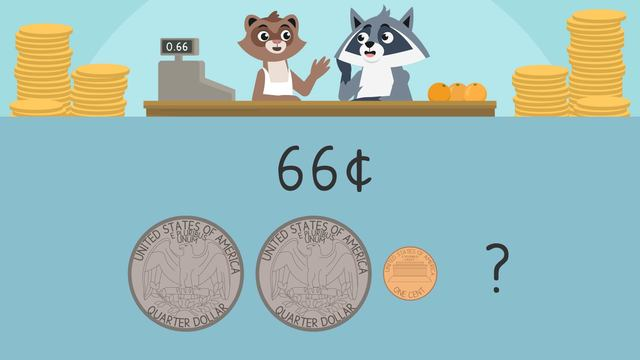# Which Coins are Missing?

Content Which Coins are Missing?Rating

Ø 5.0 / 3 ratings
The authorsTeam Digital

## Basics on the topicWhich Coins are Missing?

### In this Missing Coins Video

Freddie is getting ready to start a new job as a cashier. He is nervous about adding mixed coins in order to give change. Zuri helps him practice finding the missing coin sum and which coin values are needed to complete the change.

### US Coins

When making change, we can use a combination of these coins to make the different amounts.### How to Determine Which Coins are Missing

Many combinations of coins can be made, but we want to try and make the combination that uses the fewest coins possible.

We can determine missing coins from change given by organizing the coins from greatest to least and adding up the known coin values.Then subtract that amount from the total. Find the coin combination needed to make the amount using the fewest coins possible## Which Coins are Missing? exercise

Would you like to apply the knowledge you’ve learned? You can review and practice it with the tasks for the video Which Coins are Missing?.
• ### Match each coin with its correct value.

Hints

Remember, the penny has the lowest value and is a different color than most of the others.

Remember, the dollar coin has the highest value, and is not silver or grey.

Solution
• A dollar coin is worth \$1.00
• A half dollar coin is worth 50 cents
• A quarter is worth 25 cents
• A dime is worth 10 cents
• A nickel is worth 5 cents
• A penny is worth 1 cent
• ### Find the missing coin.

Hints

First, add up the values of the coins Freddie has.

Second, subtract the value of the coins Freddie has from 42 cents.

How many cents does Freddie still need to make 42 cents? What coin can he use?

Solution
• First add the value of the coins Freddie has, which is a quarter (25 cents), a dime (10 cents), and two pennies (2 cents). That is 37 cents.
• Next, subtract 42 - 37 = 5 cents.
• The missing coin Freddie needs is a nickel to make 42 cents change.
• ### Help Freddie make change.

Hints

This is the value of each coin.

• What is the value of 1 quarter and 1 dime?
• What other coins will you need?

After Freddie takes 1 quarter and 1 dime, subtract their value from 50 cents. This is how much more he needs.

Solution
• First, add the values of the coins given. The quarter is worth 25 cents and the dime is worth 10 cents.
• Then, subtract that value from 50 cents. (50-35=?)
• You get 15 cents. Which coins make 15 cents? One dime and one nickel.
• ### What coin is missing?

Hints

These are the values of each coin.

• How much is two quarters worth?
• What other coins will you need?

Once you find the value of two quarters, subtract that from 87 cents to figure out what else you will need.

It helps to start counting coins with the highest value.

Solution

Freddie starts with two quarters, so this is 25 + 25 = 50 cents.

Subtract 50 cents from 87 cents to figure out how much more Freddie needs: 87 - 50 = 37 cents.

You need one quarter, one dime, and two pennies to add together to make 37 cents: 25 + 10 + 1 + 1 = 37.

• ### What coin is missing?

Hints

First, add the value of the quarter and two pennies.

Subtract that value from 52 cents to find number of cents left.

What coin is the same as the value you found?

Solution

First, add the values of the coins given. The quarter is worth 25 cents and the two pennies is worth 2 cents.

Then, subtract that value from 52 cents: 52 - 27 = 25

You get 25 cents, so the missing coin is a quarter.

• ### Find pairs of coins that add up to 75 cents.

Hints

Count each set of coins.

Figure out how much is left to make 75 cents. Find the number of coins that shows that value.

Solution

These sets of coins make 75 cents:

• 1 quarter, 1 dime, and 1 nickel + 3 dimes and 1 nickel
• 2 quarters, 1 dime, and 1 nickel + 1 quarter and 1 dime
• 1 dime and 1 nickel + 2 quarters and 1 nickel
• 1 quarter + 2 quarters